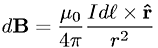# "Biot-Savart equivalent" of Faraday's Law?

• greswd
The Jefimenko equations may be consistent with the equation above, but that's not really the point of the question. The point of the question is whether the Biot-Savart Law (equation without taking into account Coulomb's Law) is consistent with the Jefimenko equations.I don't know.f

#### greswd

That thread was dominated by one verbose poster. I am repeating this thread so as to hear the opinions of others.

Ampere's Law can be derived from the Biot-Savart Law.Faraday's Law is similar to Ampere's Law.

Is there a "Biot-Savart equivalent" of Faraday's Law?

I imagine it might look something like this: (not taking into account Coulomb's Law)

$$\frac{d\vec{E}}{dV}=\frac{-\left(\frac{∂\vec{B}}{∂t}\right)×\vec{1_r}}{4\pi r^2}$$

Last edited:
Without an equation like the one above, how can we construct the entire E-vector field due to a changing magnetic field?

Maxwell's equations include ##∇×B=\mu_0 J## and ##∇×E=-\frac{∂B}{∂t}##

##∇×B=\mu_0 J## cannot adequately describe the Biot-Savart Law, the same with ##∇×E=-\frac{∂B}{∂t}##, which is why I've conceived the above.

It seems there's not much response here.
Also have you checked with your profs?
Yeah, but I don't think that this is a boring question.

Prof is asleep. No response.
The wiki doesn't have anything on this.

I thought of this question because of the parallel between E and M and I also want to construct the entire E-vector field due to a changing magnetic field

Try finding a prof who isn't asleep maybe an applied mathematics profs or one in EE.

Well, if the magnetic field is time dependent then also very likely the electric field is, and then you need (generally) the full Maxwell equations. So the Ampere law should be used as the full Ampere-Maxwell Law,
$$\vec{\nabla} \times \vec{B}-\frac{1}{c} \partial_t \vec{E}=\frac{1}{c} \vec{j}.$$
The Maxwell equations for given charge-current distributions are solved by the retarded propagator (aka Jefimenko equations):

https://en.wikipedia.org/wiki/Jefimenko's_equations

•jedishrfu
The Maxwell equations for given charge-current distributions are solved by the retarded propagator (aka Jefimenko equations):

https://en.wikipedia.org/wiki/Jefimenko's_equations

Ooh, thanks for bringing that to my attention. I had conceived Jefimenko's idea independently, now I know of the equations.

is the formula below (after taking into account Coulomb's Law) consistent with the Jefimenko equations?

$$\frac{d\vec{E}}{dV}=\frac{-\left(\frac{∂\vec{B}}{∂t}\right)×\vec{1_r}}{4\pi r^2}$$